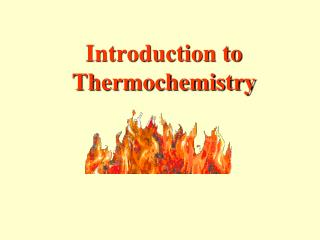# Introduction to Thermochemistry - PowerPoint PPT PresentationDownload PresentationIntroduction to Thermochemistry

Introduction to Thermochemistry
Download Presentation## Introduction to Thermochemistry

- - - - - - - - - - - - - - - - - - - - - - - - - - - E N D - - - - - - - - - - - - - - - - - - - - - - - - - - -
##### Presentation Transcript

1. Introduction to Thermochemistry

2. Chemistry Joke A Bear-y Good Pun! The vet at the zoo had a problem: beryllium. He had only two options, to curium or to barium. He lead the dentist to boron the bear’s tooth. I’d give a nickel to have seen what happened when the bear woke up. Now the vet and the dentist argon. It’s ironic what can happen when you have a beryllium and try to curium.

3. Law of Conservation of Energy • In any chemical or physical process, energy is neither created nor destroyed. • All the energy in a process (or reaction) can be accounted for as work, stored energy, or heat.

4. Heat • The energy that is transferred from one body to another because of temperature differences • Flows from warmer → cooler object • Only changes caused by heat can be detected – like changes in temperature

5. Units of Heat • Joule (J) is the SI unit of heat and energy. • calorie (cal) is another commonly used unit of energy defined as the quantity of heat needed to raise the temperature of 1g of water 1oC. • 4.184 J = 1 cal

6. Enthalpy • Heat can be represented by either q or H. • For systems at constant pressure, the heat content, q, is the same as a property of the system called enthalpy, H. • Since most reactions are carried out at constant pressure, q (heat) and H (enthalpy) are often used interchangeably. • Changes in enthalpy = H • q = H at constant pressure

7. Calorimetry - The measurement of the heat into or out of a system for either chemical or physical processes is called calorimetry. • Calorimeter—the device used to measure this absorption or release of heat

8. Foam cups are excellent heat insulators and are commonly used as simple calorimeters.

9. System vs. SurroundingsWhich way does the heat flow? • In any chemical reaction or change of physical state, heat is either released or absorbed. • In studying which direction heat flows, we use these two definitions: • the system - the part of the universe on which you focus your attention • the surroundings - includes everything else in the universe

10. Exothermic or Endothermic • Endothermic Process: Heat Absorbed • Heat going into the system from the surroundings. • Surroundings cool down. • Example: Chemical Ice Pack • q or ∆H is positive • Exothermic Process: Heat Released • Heat leaving the system and going into the surroundings. • Surroundings heat up. • Example: Combustion • q or ∆H is negative

11. Thermochemical Equations • A thermochemical equation shows the heat change. • The heat is usually given in kJ and can be included in the reaction itself or directly after the equation. • The heat change for a reaction is called Hrxn.

12. Exothermic Reactions • Energy is written as a product, or • H is negative CaO (s) + H2O(l)  Ca(OH)2 + 65.2 kJ or CaO (s) + H2O(l)  Ca(OH)2 H = -65.2 kJ

13. Endothermic Reactions • Energy is written as a reactant • H is positive 2NaHCO3 + 129 kJ Na2CO3 + H2O +CO2 or 2NaHCO3 Na2CO3 + H2O(g) +CO2 H = 129 kJ

14. Exothermic or Endothermic? Exo C2H5OH(l) + 3O2(g)  2CO2(g) + 3H2O(l) + 1235 kJ PCl5(s)  PCl3(g) + Cl2H = 87.9 kJ H2(g) + F2(g)  2HF(g) H = -536 J CO2(g) + 393.5 kJ  C(s, graphite) + O2 (g) Endo Exo Endo

15. Potential Energy Diagrams H is negative Energy is a Product H is positive Energy is a Reactant

16. Phase Changes • Physical processes can also have energy changes. • Which phase changes are exothermic and which are endothermic? • Gas → Liquid? ____________ • Liquid → Solid? ____________ • Solid → Liquid? ____________ • Liquid → Gas? _____________ Exo Exo Endo Endo

17. Phase Changes • There are special names for the heat required to melt or vaporize a substance. • The molar heat of fusion is the amount of heat required to melt one mole of a substance: Hfus. • The molar heat of vaporization is the amount of heat required to vaporize one mole of a substance: Hvap.

18. Heating Curve • A heating curve is a graph that shows phase changes in a plot of temperature vs. time. • The slanted lines show heating and an increase in kinetic energy. • The flat lines show phase changes and an increase in potential energy. (Hfus, Hvap)

19. Heating Curve Hvap Hfus a → b solid warming b → c solid becoming a liquid c → d liquid warming d → e liquid becoming a gas e → f gas warming

20. Chemistry Joke Q: Is silicon spelled the same way in Spanish? A: Si!